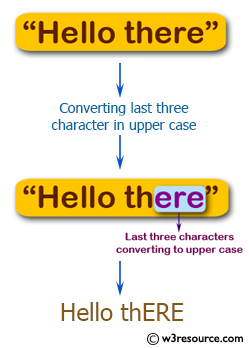﻿ Swift Basic Programming Exercise: Convert the last three characters in upper case - w3resource# Swift Basic Programming Exercise: Convert the last three characters in upper case

## Swift Basic Programming: Exercise-19 with Solution

Write a Swift program to convert the last three characters in upper case. If the string has less than 3 chars, lowercase whatever is there.

Pictorial Presentation:Sample Solution:

Swift Code:

``````func case_str(_ input: String) -> String {
if input.characters.count < 3
{
return input.lowercased()
}
else
{
var newInput = input.characters
var str1: String = ""
let lastThree = newInput.suffix(3)
newInput.removeLast(3)
let lastThreeUpper = String(lastThree).uppercased()
str1.append(contentsOf: newInput)
str1.append(String(lastThree).uppercased())
return str1
}
}
print(case_str("Hi"))
print(case_str("Hello there"))
print(case_str("Is"))
print(case_str("Python"))
```
```

Sample Output:

```hi
Hello thERE
is
PytHON
```

Swift Programming Code Editor:

Improve this sample solution and post your code through Disqus

What is the difficulty level of this exercise?

﻿